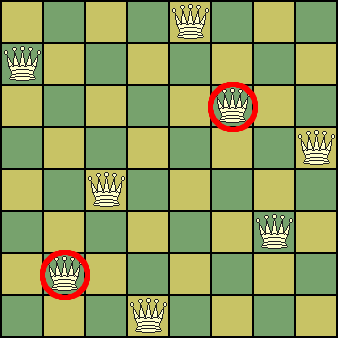#Eight Queens Puzzle

Math Lair Home > Puzzles & Problems > Eight Queens Puzzle

A classic chess-related problem (although it doesn't require much if any knowledge of the rules of chess) is the eight Queens problem. The puzzle can be stated simply: Place 8 Queens on a chessboard such that no two are attacking each other. Since chess Queens move any number of spaces along any row, column, or diagonal, the puzzle could also be stated as: place eight Queens (or any other items) on an 8×8 board such that no two are in the same row, column or diagonal.The diagram on the right shows an incorrect solution to the problem. Note that the two Queens circled in red are attacking each other, making the solution incorrect. Can you find a correct solution to the problem?

Here are some hints if you're stumped. The problem is in fact possible. There are 12 unique solutions. Including rotations and reflections, there are 92 solutions. It may help to attack the problem in an organized fashion. Here are some possible ideas:

• Put one Queen in each row and column, and then adjusting pairs of Queens until none are in the same diagonal.
• Put all of the Queens in, say, the bottom row, move the leftmost Queen to any square, and then, starting in the second column from the left, advance each Queen until it is on a square that is not in the same row, column, or diagonal as any other. If you can't find a solution, start over, and move the leftmost Queen somewhere else.
• Try placing as many Queens as possible a Knight's move away from one another (Knights have an "L"-shaped move, two squares in one direction and one in the other direction; two Queens a Knight's move away from each other cannot be in the same row, column, or diagonal).

One solution can be found on the answers page.

This puzzle is also known as the eight checkers puzzle, with the object being to place eight checkers on a checkerboard such that no two lie in the same row, column, or diagonal. The problem, and the solution, are the exact same.